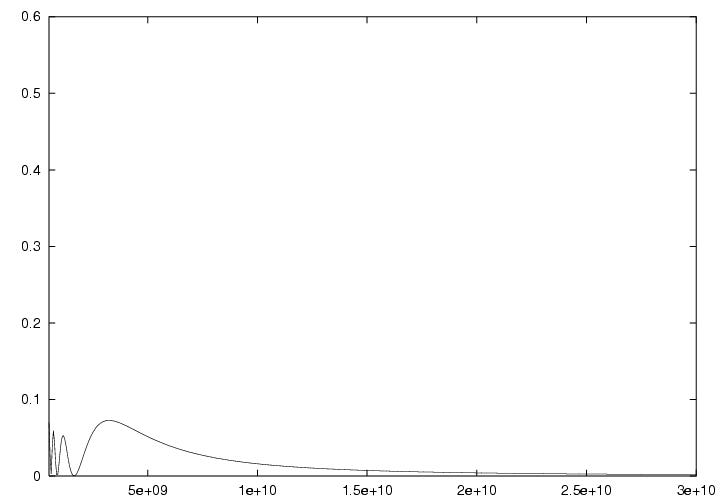# The Globe and Neutrino Oscillations

This page includes a sample of neutrino oscillation probabilites for different baselines in the Earth. The page requires java script enabled at your browser to work.

The following calculations assume a spherically symmetrical Earth with radius 6368 km and a density profile acquired from the PREM -model. It is assumed that the neutrino source is at 0 degrees of latitude and 0 degrees longitude and that the detector is at 0 degrees of latitude and (long.) degrees of longitude, both on the surface of Earth.

The columns are as follows:

• (long.) The longitude of the target station.
• (dist.) Baseline distance.
• (1) Muon neutrino to electron neutrino oscillation probability (normal mass hierarchy)
• (2) Antimuon neutrino to antielectron neutrino oscillation probability (normal mass hierarchy)
• (3) Muon neutrino to electron neutrino oscillation probability (inverted mass hierarchy)
• (4) Antimuon neutrino to antielectron neutrino oscillation probability (inverted mass hierarchy)
In calculating the plots, following parameters were used:
delta m322 = ± 3.5e-3
delta m212 = +7e-5
sin22t12 = 0.87
sin22t23 = 1.0
sin22t13 = 0.1
delta = 0

By rolling the mouse over the 'X's the oscillation probability vs. E -plot changes correspondingly. The x-axis is the neutrino energy (from 500 MeV to 30 GeV) and y-axis is the oscillation probability. Clicking the 'X' returns the page to the picture. Clicking the distances will open a new window with a plot of the potential profile (in electron volts) as a function of the distance (in meters) for the corresponding baseline.

long. dist. 1 2 3 4 long. dist. 1 2 3 4 long. dist. 1 2 3 45 o 556 km X X X X 65 o 6843 km X X X X 125 o 11297 km X X X X 10 o 1110 km X X X X 70 o 7305 km X X X X 130 o 11543 km X X X X 15 o 1662 km X X X X 75 o 7753 km X X X X 135 o 11767 km X X X X 20 o 2212 km X X X X 80 o 8187 km X X X X 140 o 11968 km X X X X 25 o 2757 km X X X X 85 o 8604 km X X X X 145 o 12147 km X X X X 30 o 3296 km X X X X 90 o 9006 km X X X X 150 o 12302 km X X X X 35 o 3830 km X X X X 95 o 9390 km X X X X 155 o 12434 km X X X X 40 o 4356 km X X X X 100 o 9756 km X X X X 160 o 12543 km X X X X 45 o 4874 km X X X X 105 o 10104 km X X X X 165 o 12627 km X X X X 50 o 5382 km X X X X 110 o 10433 km X X X X 170 o 12688 km X X X X 55 o 5881 km X X X X 115 o 10741 km X X X X 175 o 12724 km X X X X 60 o 6368 km X X X X 120 o 11030 km X X X X 180 o 12734 km X X X X

For any comments, email Juho Sarkamo.

Back to mainpage